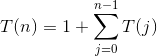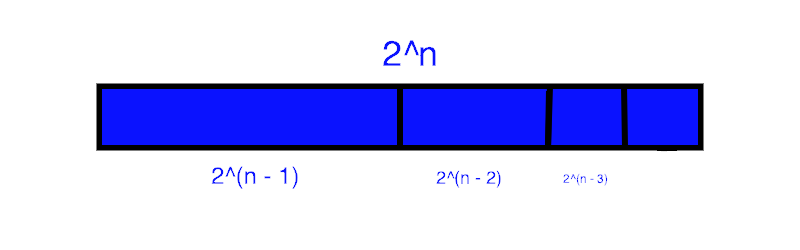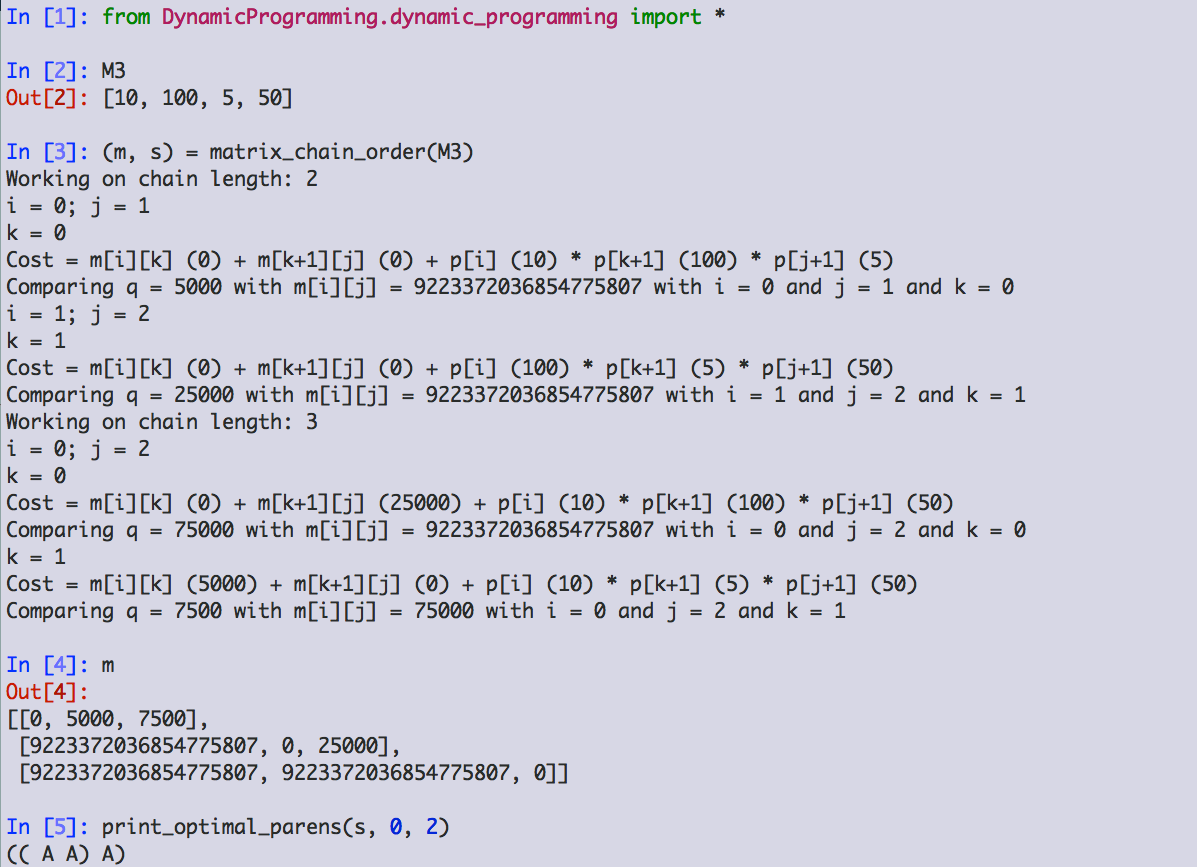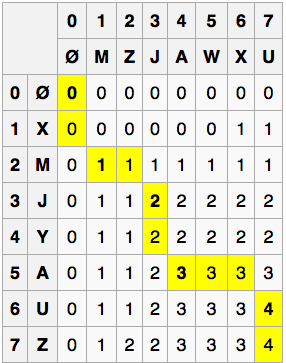# Design and Analysis of Algorithms: Dynamic ProgrammingWhat is dynamic programming?

Memoization!

The name is largely a marketing construct. Here is the inventor of the term, Richard Bellman, on how it came about:

"I spent the Fall quarter (of 1950) at RAND. My first task was to find a name for multistage decision processes. An interesting question is, Where did the name, dynamic programming, come from? The 1950s were not good years for mathematical research. We had a very interesting gentleman in Washington named Wilson. He was Secretary of Defense, and he actually had a pathological fear and hatred of the word research. I'm not using the term lightly; I'm using it precisely. His face would suffuse, he would turn red, and he would get violent if people used the term research in his presence. You can imagine how he felt, then, about the term mathematical. The RAND Corporation was employed by the Air Force, and the Air Force had Wilson as its boss, essentially. Hence, I felt I had to do something to shield Wilson and the Air Force from the fact that I was really doing mathematics inside the RAND Corporation. What title, what name, could I choose? In the first place I was interested in planning, in decision making, in thinking. But planning, is not a good word for various reasons. I decided therefore to use the word "programming". I wanted to get across the idea that this was dynamic, this was multistage, this was time-varying. I thought, let's kill two birds with one stone. Let's take a word that has an absolutely precise meaning, namely dynamic, in the classical physical sense. It also has a very interesting property as an adjective, and that it's impossible to use the word dynamic in a pejorative sense. Try thinking of some combination that will possibly give it a pejorative meaning. It's impossible. Thus, I thought dynamic programming was a good name. It was something not even a Congressman could object to. So I used it as an umbrella for my activities."
(Source: https://en.wikipedia.org/wiki/Dynamic_programming#History)

Note that Bellman's claim that "dynamic" can be use pejoratively is surely false: most people would not favor "dynamic ethnic cleansing"!

Algorithms that use dynamic programming:
• Recurrent solutions to lattice models for protein-DNA binding
• Backward induction as a solution method for finite-horizon discrete-time dynamic optimization problems
• Method of undetermined coefficients can be used to solve the Bellman equation in infinite-horizon, discrete-time, discounted, time-invariant dynamic optimization problems
• Many string algorithms including longest common subsequence, longest increasing subsequence, longest common substring, Levenshtein distance (edit distance)
• Many algorithmic problems on graphs can be solved efficiently for graphs of bounded treewidth or bounded clique-width by using dynamic programming on a tree decomposition of the graph.
• The Cocke-Younger-Kasami (CYK) algorithm which determines whether and how a given string can be generated by a given context-free grammar
• Knuth's word wrapping algorithm that minimizes raggedness when word wrapping text
• The use of transposition tables and refutation tables in computer chess
• The Viterbi algorithm (used for hidden Markov models)
• The Earley algorithm (a type of chart parser)
• The Needleman-Wunsch algorithm and other algorithms used in bioinformatics, including sequence alignment, structural alignment, RNA structure prediction
• Floyd's all-pairs shortest path algorithm
• Optimizing the order for chain matrix multiplication
• Pseudo-polynomial time algorithms for the subset sum, knapsack and partition problems
• The dynamic time warping algorithm for computing the global distance between two time series
• The Selinger (a.k.a. System R) algorithm for relational database query optimization
• De Boor algorithm for evaluating B-spline curves
• Duckworth-Lewis method for resolving the problem when games of cricket are interrupted
• The value iteration method for solving Markov decision processes
• Some graphic image edge following selection methods such as the "magnet" selection tool in Photoshop
• Some methods for solving interval scheduling problems
• Some methods for solving the travelling salesman problem, either exactly (in exponential time) or approximately (e.g. via the bitonic tour)
• Recursive least squares method
• Beat tracking in music information retrieval
• Adaptive-critic training strategy for artificial neural networks
• Stereo algorithms for solving the correspondence problem used in stereo vision
• Seam carving (content-aware image resizing)
• The Bellman-Ford algorithm for finding the shortest distance in a graph
• Some approximate solution methods for the linear search problem
• Kadane's algorithm for the maximum subarray problem
Dynamic programming video
Rod cuttingNothing special here about steel rods: the algorithm applies to any good that can be sub-divided, but only in multiples of some unit, like lumber, or meat, or cloth.

Recursive top-down implmentation

Keeps calculating the same cuts again and again, much like naive, recursive Fibonacci.

Running time is exponential in n. Why?
Our textbook gives us the equation:This is equivalent to:
T(n) = 1 + T(n - 1) + T(n - 2) + ... T(1)
For n = 1, there are 20 ways to solve the problem.
For n = 2, there are 21 ways to solve the problem.
For n = 3, there are 22 ways to solve the problem.
Each additional foot of rod gives us 2 * (previous number of ways of solving problem), since we have all the previous solutions, either with a cut of one foot for the new extension, or without a cut there. (Similar to why each row of Pascal's triangle gives us the next power of two.)So, we have the series:
2n - 1 + 2n - 2 + 2n - 3... + 20 + 1
And this equals 2n. Why?Example: 24 = 23 + 22 + 21 + 20 + 1
Or, 16 = 8 + 4 + 2 + 1 + 1

Using dynamic programming for optimal rod-cutting

Much like we did with the naive, recursive Fibonacci, we can "memoize" the recursive rod-cutting algorithm and achieve huge time savings.

That is an efficient top-down approach. But we can also do a bottom-up approach, which will have the same run-time order but may be slightly faster due to fewer function calls. (The algorithm uses an additional loop instead of recursion to do its work.)

Subproblem graphsThe above is the Fibonacci sub-problem graph for fib(5). As you can see, F5 must solve F4 and F3. But F4 must also solve F3. It also must solve F2, which F3 must solve as well. And so on.

This is the sort of graph we want to see if dynamic programming is going to be a good approach: a recursive solution involves repeatedly solving the same problems.

This is quite different than, say, a parser, where the code sub-problems are very unlikely to be the same chunks of code again and again, unless we are parsing the code of a very bad programmer who doesn't understand functions!

Reconstructing a solution

In this section, we see how to record the solution we arrived at, rather than simply return the optimal revenue possible. The owner of Serling Enterprises will surely be much more pleased with this code than the earlier versions.

Run the Python code

In the console below, type or paste:
``` !git clone https://gist.github.com/80d2a774f08f686f675f8a9254570da0.git cd 80d2a774f08f686f675f8a9254570da0 from dynamic_programming import * ```

Now let's run our ext_bottom_up_cut_rod() code. (Link to full source code below.) Type or paste: ``` p4 (revs, cuts, max_rev) = ext_bottom_up_cut_rod(p4, 4) ```

You can explore more, by designing your own price arrays! Just type in:
``` my_name = [x, y, z...] ```
where 'my_name' is whatever name you want to give your price array, and x, y, z, etc. are the prices for a cut of length 1, 2, 3, etc.

A video on rod cutting
Matrix-chain multiplicationThere are many ways to parenthesize a series of matrix multiplications. For instance, if we are parenthesizing A1 * A2 * A3 * A4, we could parenthesize this in the following ways:

(A1 (A2 (A3 A4)))
(A1 ((A2 A3) A4))
((A1 A2) (A3 A4))
((A1 (A2 A3)) A4)
(((A1 A2) A3) A4)

Which way we choose to do so can make a huge difference in run-time!

Why is this different than rod cutting? Think about this for a moment, and see if you can determine why the problems are not the same.

The reason

In rod cutting, a cut of 4-2-2 is the same cut as a cut of 2-2-4, and the same as a cut of 2-4-2.
That is not at all the case for matrix parenthesization.

Counting the number of parenthesizations

The number of solutions is exponential in n, thus brute-force is a bad technique for solving this problem.

Applying dynamic programming
Step 1: The structure of an optimal parenthesization

For any place at level n where we place parentheses, we must have optimal parentheses at level n + 1. Otherwise, we could substitute in the optimal n + 1 level parentheses, and level n would be better!
Cut-and-paste proof.

Step 2: A recursive solution

If we know the optimal place to split A1... An (call it k), then the optimal solution is that split, plus the optimal solution for A1... Ak and the optimal solution for Ak+1... An. Since we don't know k, we try each possible k in turn, compute the optimal sub-problem for each such split, and see which pair of optimal sub-problems yields the optimal (minimum, in this case) total.

"For example, if we have four matrices ABCD, we compute the cost required to find each of (A)(BCD), (AB)(CD), and (ABC)(D), making recursive calls to find the minimum cost to compute ABC, AB, CD, and BCD. We then choose the best one." (https://en.wikipedia.org/wiki/Matrix_chain_multiplication)

An easy way to understand this:
Let's say we need to get from class at NYU Tandon to a ballgame at Yankee Stadium in the Bronx as fast as possible. If we choose Grand Central Station as the optimal high-level split, we must also choose the optimal ways to get from NYU to Grand Central, and from Grand Central to Yankee Stadium. It won't do to choose Grand Central, and then walk from NYU to Grand Central, and CitiBike from Grand Central to Yankee Stadium: there are faster ways to do each sub-problem!

Step 3: Computing the optimal costs

CLRS does not offer a recursive version here (they do later in the chapter); they go straight to the bottom-up approach of storing each lowest-level result in a table, avoiding recomputation, and then combine those lower-level results into higher-level ones. The indexing here is very tricky and hard to follow in one's head, but it is worth trying to trace out what is going on by following the code. I have as usual included some print statements to help.

Step 4: Constructing an optimal solution

Finally, we use the results computed in step 3 to actually provide the optimal solution, by actually determing where the parentheses go.

Here is the code from our textbook, implemented in Python, runnning on the example where A1 is 10 x 100, A2 is 100 x 5, and A3 is 5 x 50:The actual output of our Python code.

The structure of m:

 0 5000 7500 ∞ 0 25000 ∞ ∞ 0
Memoization

We can memoize the recursive version and change its run time from Ω(2n) to O(n3).

A video on matrix chains.
Elements of dynamic programming
Optimal substructure

A problem exhibits optimal substructure if an optimal solution to the problem contains within it optimal solutions to subproblems.

Overlapping subproblems

The problem space must be "small," in that a recursive algorithm visits the same sub-problems again and again, rather than continually generating new subproblems. The recursive Fibonacci is an excellent example of this!

Reconstructing an optimal solution

Storing our choices in a table as we make them allows quick and simple reconstruction of the optimal solution.

Memoization

As mentioned above, recursion with memoization is often a viable alternative to the bottom-up approach. Which to choose depends on several factors, one of which being that a recursive approach is often easier to understand. If our algorithm is going to handle small data sets, or not run very often, a recursive approach with memoization may be the right answer.

Longest common subsequence
Step 1: Characterizing a longest common subsequence

'Let X be "XMJYAUZ" and Y be "MZJAWXU". The longest common subsequence between X and Y is "MJAU".' (https://en.wikipedia.org/wiki/Longest_common_subsequence_problem)Brute force solution runs in exponential time: not so good!

But the problem has an optimal substructure:

X = gregorsamsa
Y = reginaldblack
LCS: regaa
Our match on the last 'a' is at position X11 and Y11. The previous result string ('rega') must have been the LCS before X11 and Y11: otherwise, we could substitute in that actual LCS for 'rega' and have a longer overall LCS.

Step 2: A recursive solution

Caution: here some sub-problems are ruled out! If Xi and Yj are different, we consider the sub-problems of finding the LCS for Xi and Yj - 1 and for Xi - 1 and Yj, but not for Xi and Yj. Why not? Well, if they aren't equal, they can't be the endpoint of an LCS.

Step 3: Computing the length of an LCS

The solution here proceeds much like the earlier ones: find an LCS in a bottom-up fashion, using tables to store intermediate results and information for reconstructing the optimal solution.

Step 4: Constructing an LCS
Improving the code

We could eliminate a table here, reduce aymptotic run-time a bit there. But is the code more confusing? Do we lose an ability (reconstructing the solution) we might actually need later?

An important principle: Don't optimize unless it is needed!

Video on LCS
Optimal binary search treesStep 1: The structure of an optimal binary search tree

If a binary search tree is optimally construted, then both its left and right sub-trees must be optimally constructed. The usual "cut-and-paste" argument applies.

Step 2: A recursive solution

As usual, this is straightforward, but too slow.

Step 3: Computing the expected search cost

Very much like the matrix-chain-order code. Working code coming soon!

Optimal binary search tree video
Source Code
For Further Study
Homework
1. Change memoized-rod-cut to return a list of cuts to make, instead of the maximum possible revenue. Pseudo-code or real code are both fine.
2. For the following table, determine the cost and structure of an optimal binary search tree:

i 0 1 2 3 4 5
pi .05 .05 .25 .05 .05
qi .05 .15 .05 .05 .05 .20